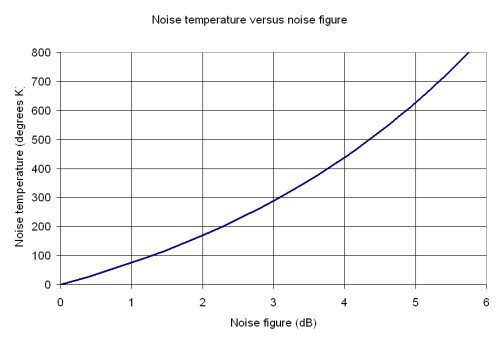# Noise Temperature

Please feel free to contribute to this humble page, like Gene did!

Noise temperature is another way of expressing signal-to-noise degradation, and is often used in radio astronomy.

T=noise temperature=290*(F-1)

Here, F is the noise factor (not noise figure). The "290" in the expression is used to denote a standardized temperature in Kelvin, which in this case is close to room temperature (290 K is an IEEE standard). We have seen "T" expressed as TN, or Te (equivalent noise temperature). Note that noise temperature of an amplifier is not directly related to the temperature of an amplifier. Below is a plot of noise temperature versus noise figure. Perhaps if you want to remember a few points to impress your friends remember that 1 dB NF is about 75 Kelvin, and 3 dB is 288 Kelvin (nearly room temperature). Note that is incorrect to say "degrees Kelvin", as Kelvin is a base unit in the SI system.Noise temperature is used mainly in radio astronomy. Emerging applications of microwave and millimeterwave radiometers have made it important for many of us all to be able to switch back and forth between the two scales.

To convert from one to the other:

Noise temperature (T) = 290 * (10^(NF/10)-1) (Kelvin)

While we're on the subject,

Noise figure (NF) = 10 * log (noise factor) dB

Regarding our rule of thumb about noise figure of a passive device (#56 last time we checked)... Gene sent in these comments. Thanks!

Linear passive devices have noise figure equal to their loss. Expressed in dB, the NF is equal to -S21(dB). Something with one dB loss has one dB noise figure.

May I suggest a more refined definition of this rule? This statement is true only if the passive linear device is at room temperature. However, if it is at a different physical temperature than room temperature (or To for that matter), the noise figure will be different. If I did my calculation properly, I believe that the noise figure would be

F = 1+(1/G-1)*Tp/To

Where G is the gain of the device (less than or equal to 1), and Tp is the physical temperature of the device. Therefore, I would recommend that the statement should say, "Linear passive devices at room temperature have a noise figure equal to their loss. Expressed in dB, the NF is equal to -S21(dB). Something with one dB loss has one dB noise figure at room temperature." I know that the NF wouldn't change very much if the device is at a physical temperature near room temperature, but if some poor slob is working at temperatures very different than room temperature, their assumption that the NF would be equal to the loss would be incorrect.

Author : Unknown Editor